## Find Zeros, Poles, and Gains for CTLE from Transfer Function

This example shows how to get a set of zeros, poles and gains from a transfer function and use these to configure the Specification parameter `GPZ Matrix` of a CTLE in the SerDes Designer app. You can convert the poles and residues output by the `rationalfit` function into a set of zeros, poles, and gains. You can then reformat the set of zeros, poles, and gains to use as a `GPZ matrix` in a CTLE block.

### Import Transfer Function

Import a .csv file containing a transfer function using the function `readmatrix.`

```ctle_transfunc = readmatrix('transfer_function.csv','Range','A7:C775'); freq = ctle_transfunc(:,1); ri = ctle_transfunc(:,2:end);```

### Convert Transfer Function to Complex Form

To prepare data for use by `rationalfit`, convert the real numbers from the transfer function to complex numbers using `complex` function.

`data = complex(ri(:,1:2:end),ri(:,2:2:end));`

### Find Rational Fit of Transfer Function

Set the number of poles to `8` for use by `rationalfit`. Then use a loop function to model the complex transfer function using `rationalfit.` Plot data to evaluate the results from `rationalfit.`

```npoles = 8; fit = rfmodel.rational; errdb = zeros(size(npoles)); %find rational fit [fit(npoles),errdb(npoles)] = rationalfit(freq,data(:,1),'NPoles',npoles,'IterationLimit',100); %calculate the frequency-response of array "fit" using array "freq" resp(:,npoles) = freqresp(fit(npoles),freq); figure %plot each point of array "data" and each value of array "resp" to compare: plot(freq,abs(data(:,1)),'-o',freq,abs(resp(:,npoles)))```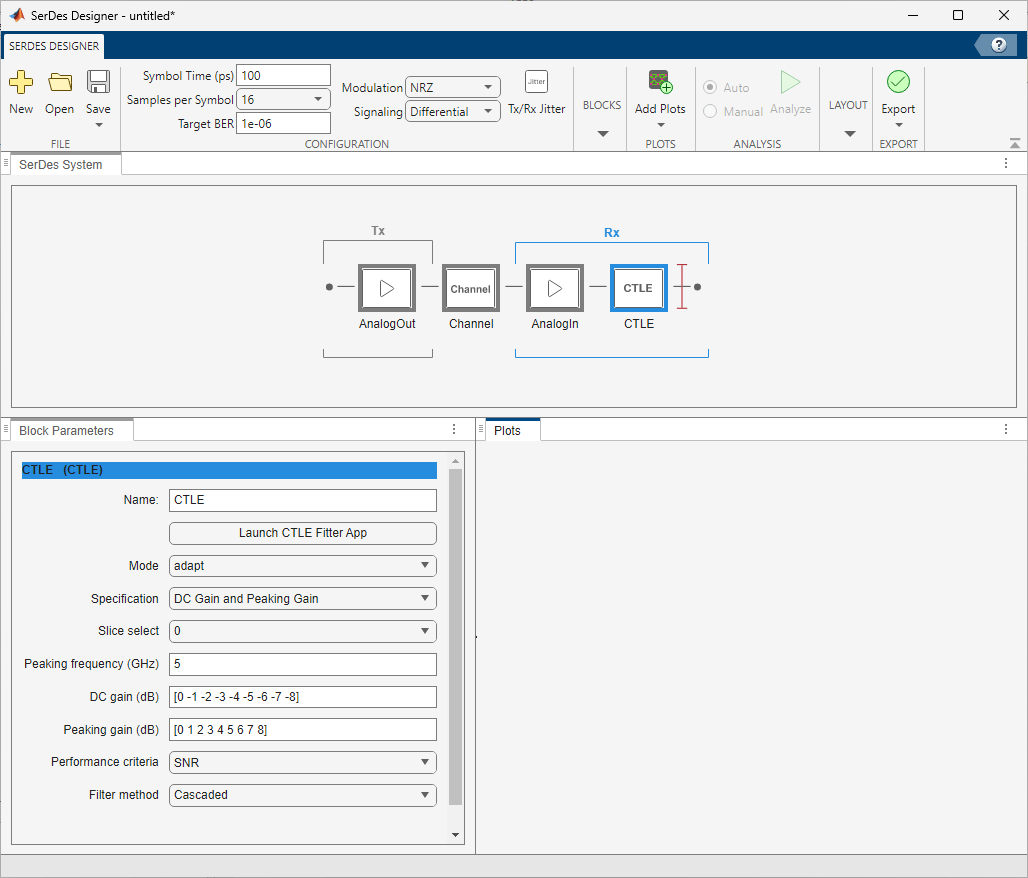```%title(sprintf('npoles %d errdb %g',npoles,errdb(npoles))) [besterrdb,bestindex] = min(errdb); bestfit = fit(bestindex); bestresp = resp(:,bestindex); dt = 1/(16*freq(end)); T = (0:499)*dt;```

### Convert to Zeros, Poles, Gains from Poles and Residues

`rationalfit` returns poles and residues, but you need to convert these into zeros, poles and gains. The CTLE can be configured to use Specification parameter "`GPZ Matrix`" where the units for gains, poles and zeros are dB, Hz, and Hz, respectively.

```gpz = zeros(1,2*max(npoles)); [g1, p, z, gs] = GPZFromRationalFit(bestfit(1)); gpz(1,1) = g1; gpz(1,2:2:length(p)*2) = p; gpz(1,3:2:length(z)*2+1) = z; ```

### Configure CTLE Block in SerDes Designer

Launch `serdesDesigner`. Place a CTLE at the RX. Set the CTLE to use `GPZ Matrix` from the Specification parameter in the Block Parameters tab for CTLE. Then copy the contents of `gpz` above and paste into the entry for Gain pole zero matrix.

`serdesDesigner; `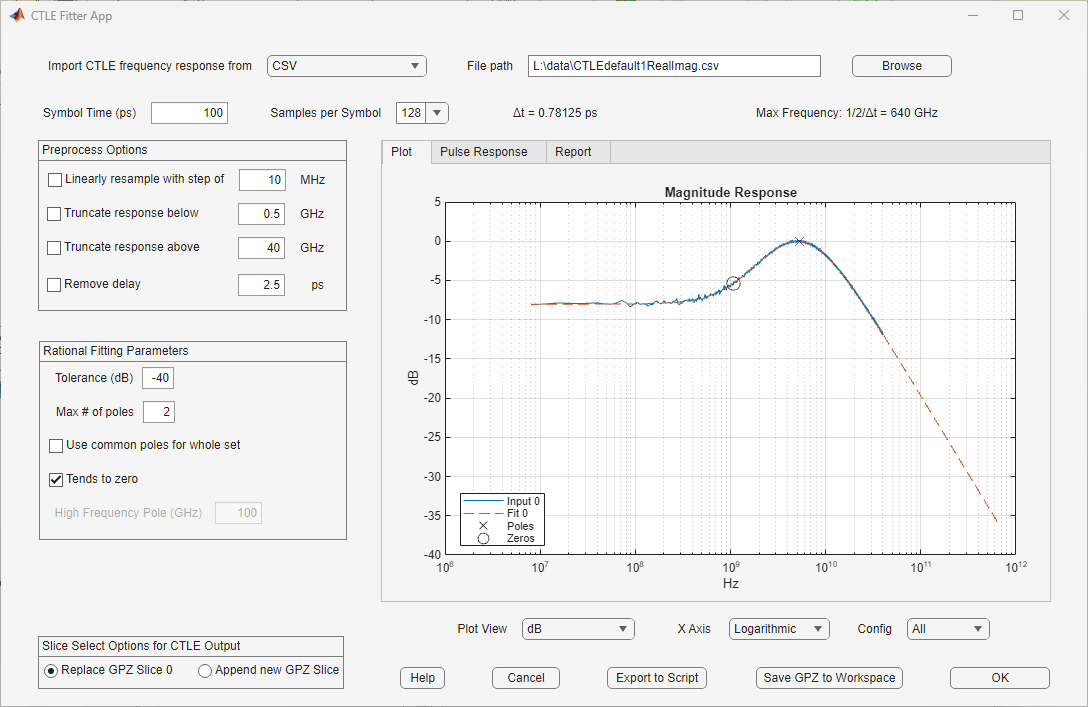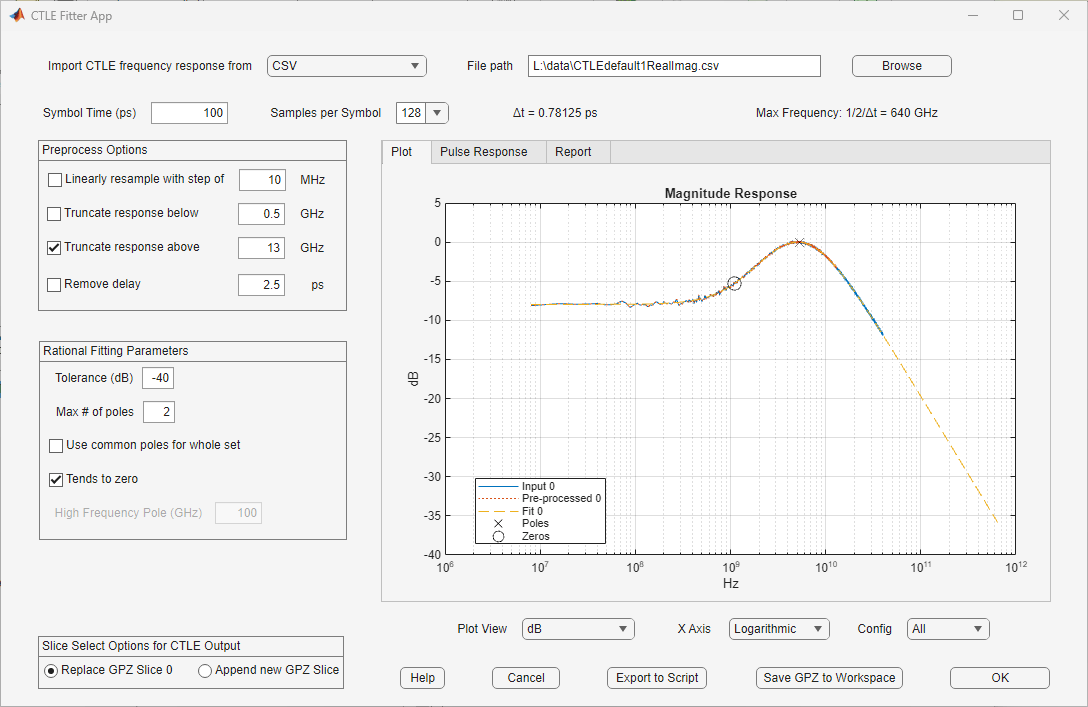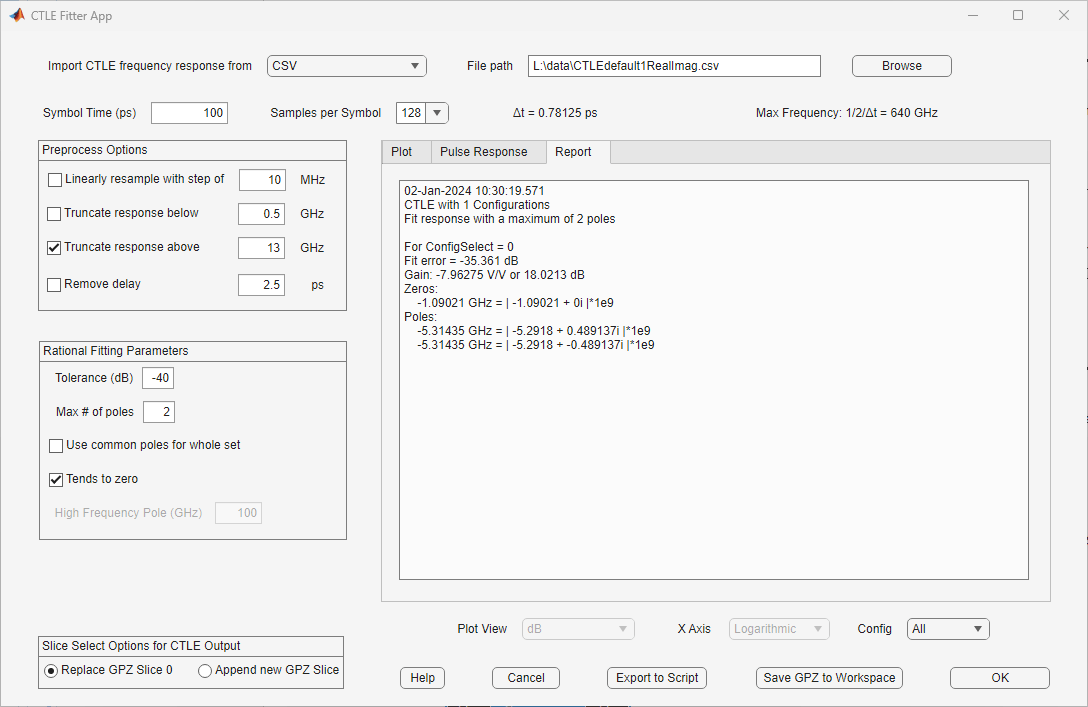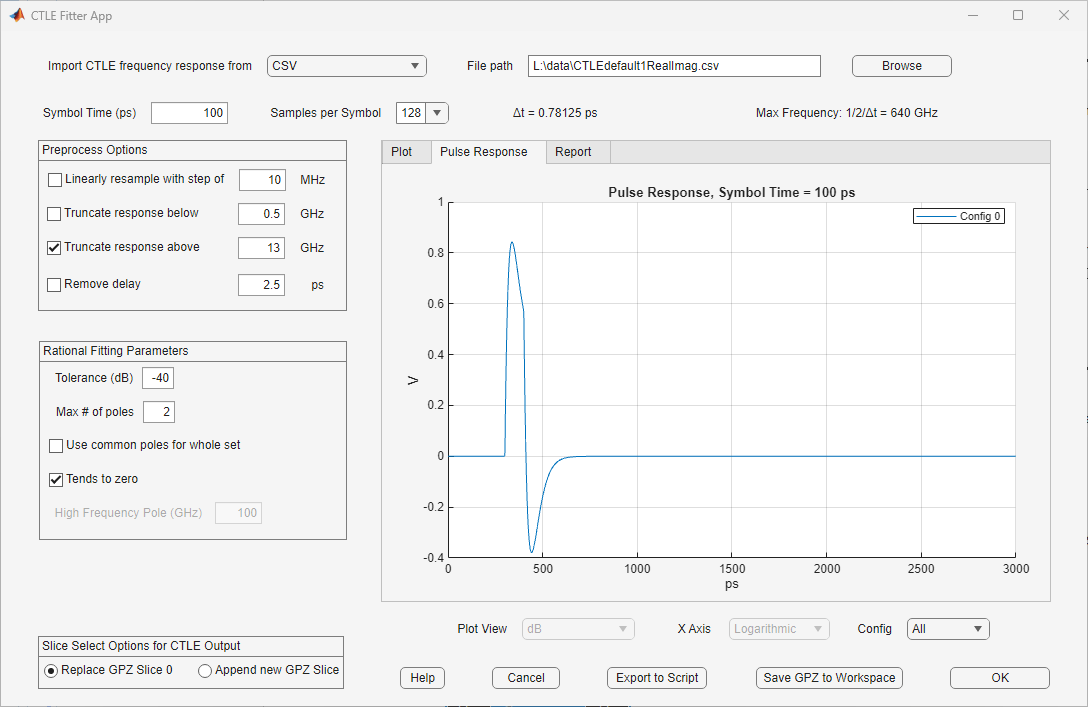### Corellate Pulse Response in SerDes Designer to IBIS-AMI Simulation

Within the serdesDesigner App, plot the Pulse Response. Then Export->Make IBIS AMI Model for SerDes System. The IBIS-AMI model may be loaded into an appropriate EDA tool to plot the Pulse Response from the model. Plots for Pulse Response from the App and the EDA tool may be compared for corellation purposes.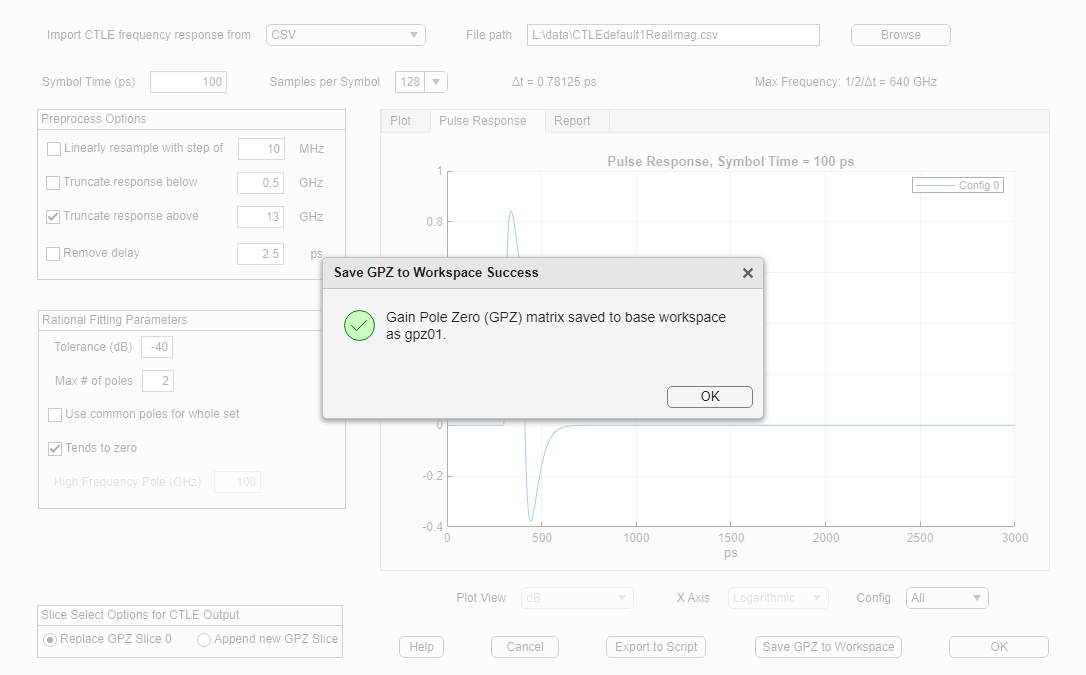##### SupportGet trial now